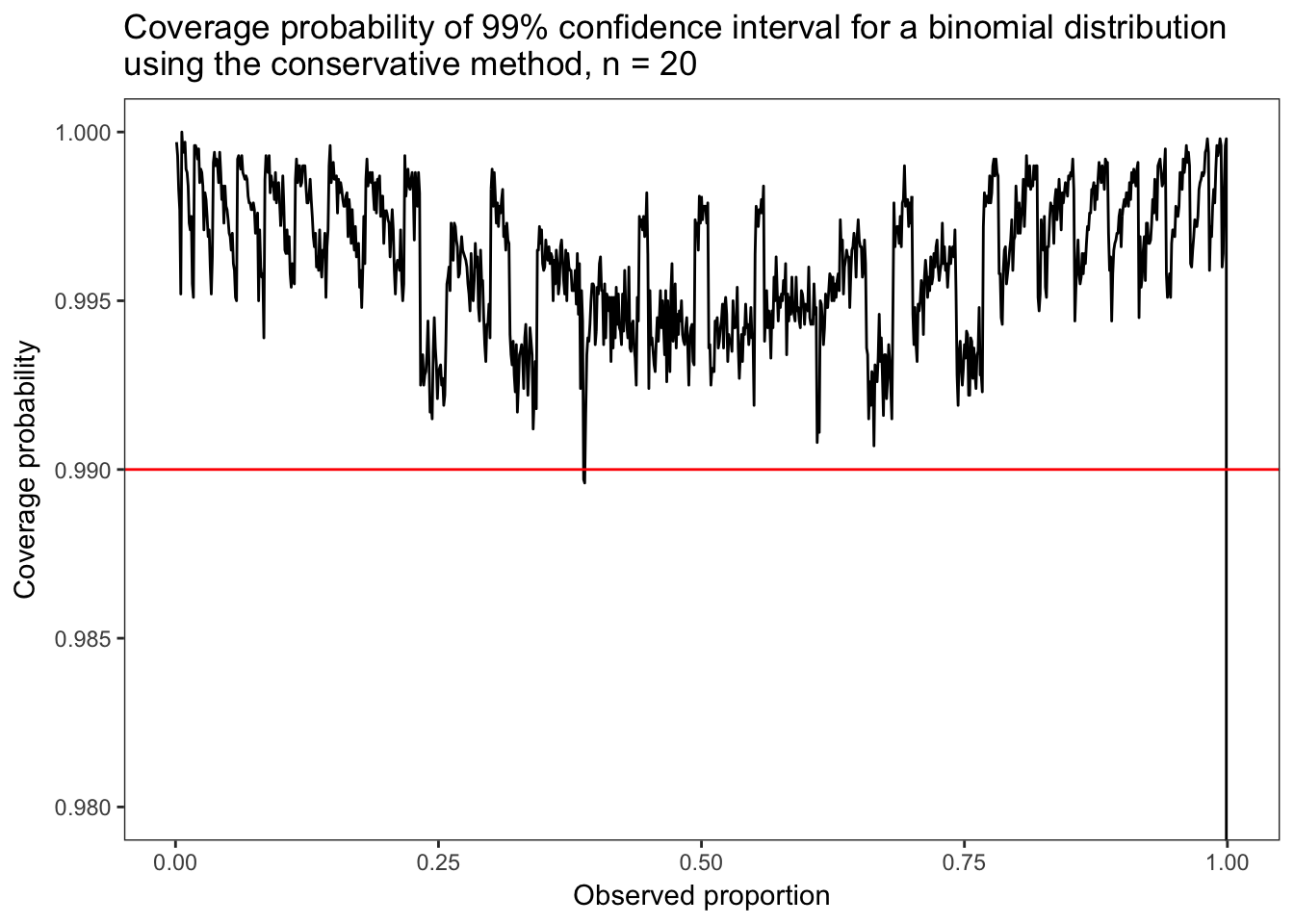The binomial distribution is good for modeling binary outcomes like sales lead hits / misses, manufacturing defects, or just about any process that can be viewed as having two possible outcomes (caveat: w/ replacement; for w/o replacement see the hypergeometric distribution).

Because so many actual business processes fit the binary mold, the binomial distribution is a very useful distribution.

Statisticians think a lot about the failure cases of their tools and ways to minimize those cases (high quality intervals). This is my follow-along exploration of the book, Statistical Intervals, written by my Masters advisor Luis Escobar and his colleagues William Meeker and Gerald Hahn. I highly recommend it.

suppressPackageStartupMessages({
suppressWarnings(invisible(
sapply(c("purrr", "dplyr", "ggplot2", "ggthemes"),
function(x) { library(x, character.only = TRUE) })))
}) 

I’m going to think of an unfair coin with a particular chance of heads > 50%. Let’s say, 0.6327543. Now I’m going to flip the coin 38 times while recording the outcomes. From the outcomes, I’d like construct some 99% confidence intervals for $$\hat{p}$$.

p # <- runif(1, 0.5, 1)
##  0.6327543
flips # <- sample(seq_len(100), 1)
##  38
(outcomes <- rbinom(flips, size = 1, prob = p))
##   1 0 1 0 0 0 1 1 1 1 0 1 0 1 0 0 1 0 0 1 0 1 1 1 1 1 0 1 1 1 1 1 0 0 0
##  1 0 1

We can estimate $$\hat{p}$$ by $$\frac{x}{n}$$, 0.5789474.

# The conservative method for binomial confidence intervals.

alpha <- 1 - 0.99
(lower <- qbeta(alpha / 2, sum(outcomes), length(outcomes) - sum(outcomes) + 1))
##  0.3608022
sum(outcomes)/length(outcomes)
##  0.5789474
(upper <- qbeta(1 - alpha / 2, sum(outcomes) + 1, length(outcomes) - sum(outcomes)))
##  0.7769871

Now I’d like to examine the coverage probability of this proported conservative method. To do that, first I’ll make a handy function for calculating a single interval,

conservative_interval <- function(outcomes, alpha) {
c(qbeta(alpha / 2, sum(outcomes), length(outcomes) - sum(outcomes) + 1),
qbeta(1 - alpha / 2, sum(outcomes) + 1, length(outcomes) - sum(outcomes)))
}
conservative_interval(outcomes, alpha)
##  0.3608022 0.7769871

Coverage probability is the proportion of times intervals constructed by the method will tend to contain the true value. Basically, if the interval is listed as a 99% confidence interval, how will it actually perform?

times <- replicate(1e4, conservative_interval(rbinom(flips, 1, p), alpha))
times[, 1:3] # let's peek at the first three intervals
##           [,1]      [,2]      [,3]
## [1,] 0.3856211 0.3608022 0.4906330
## [2,] 0.7980822 0.7769871 0.8765353

Now I’d like to calculate the coverage probability of this method or the proportion of times the true proportion is contained by the interval.

purrr::map(seq_len(ncol(times)), function(i) { times[1, i] < p & p < times[2, i] }) %>%
keep(~ .x) %>% unlist() %>%
{ sum(.) / ncol(times) }
##  0.9949

As expected, the “conservative” confidence interval yields a coverage probability greater than the 99% label. Now let’s look over a range of observed proportions for a given size,

flips <- 20
# beware this is a small step size! might want to start with 0.01.
parallel::mclapply(seq(0.001, 1, 0.001), function(p) { # might want purrr::map if memory consumption is too much!
times <- replicate(1e4, conservative_interval(rbinom(flips, 1, p), alpha = 0.01))
purrr::map(seq_len(ncol(times)), function(i) { times[1, i] < p & p < times[2, i] }) %>%
keep(~ .x) %>% unlist() %>%
{ sum(.) / ncol(times) } %>%
tibble(coverage_probability = .) %>%
mutate(observed_proportion = p)
}, mc.cores = parallel::detectCores()) %>%
bind_rows() %>%
ggplot(aes(x = observed_proportion, y = coverage_probability)) +
geom_line() +
geom_hline(yintercept = 0.99, colour = "red") +
coord_cartesian(ylim = c(0.98, 1)) +
theme_bw() +
ylab("Coverage probability") +
xlab("Observed proportion") +
theme(panel.grid = element_blank()) +
ggtitle("Coverage probability of 99% confidence interval for a binomial distribution\nusing the conservative method, n = 20")Great! It looks like the conservative method is conservative as it almost never (extremely rarely?) dips below the listed confidence interval level.

The following is a handy graph of the conservative method 99% confidence interval for various sample sizes,

step <- 0.00001 # very small step size! beware!
observed_proportion <- seq(0 + step, 1 - step, step)
parallel::mclapply(c(2, 3, 5, 10, 25, 50, 100, 1000), function(number_of_observations) {
map(observed_proportion, function(x) {
conservative_interval(rbinom(number_of_observations, 1, x), 0.01) }) %>%
{ bind_cols(map(., function(x) { x }) %>% unlist() %>% tibble(lower = .),
map(., function(x) { x }) %>% unlist() %>% tibble(upper = .)) %>%
mutate(observed_proportion, number_of_observations) }
}, mc.cores = parallel::detectCores()) %>%
bind_rows() %>%
ggplot(aes(x = observed_proportion, colour = factor(number_of_observations))) +
geom_smooth(aes(y = lower), method = "gam", formula = y ~ s(x, bs = "ps"),
se = FALSE, size = 1) +
geom_smooth(aes(y = upper), method = "gam", formula = y ~ s(x, bs = "ps"),
se = FALSE, size = 1) +
coord_cartesian(xlim = c(0, 1), ylim = c(0, 1)) +
scale_x_continuous(breaks = seq(0, 1, 0.1)) +
scale_y_continuous(breaks = seq(0, 1, 0.1)) +
scale_color_colorblind(name = "Number of observations", guide = guide_legend(override.aes = list(size = 3))) +
theme_bw() +
theme(panel.grid.major = element_line(color = "black", size = 0.2),
panel.grid.minor = element_line(color = "black", size = 0.2)) +
ylab("Conservative interval") +
xlab("Observed proportion") +
ggtitle("99% conservative confidence interval for a binomial proportion")